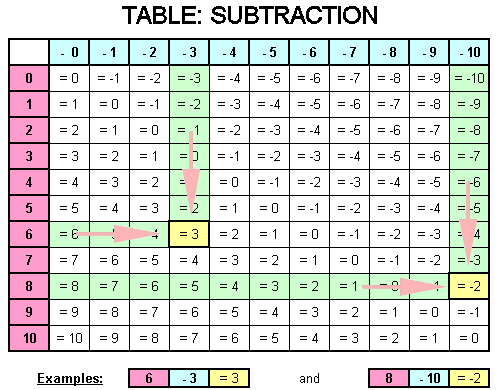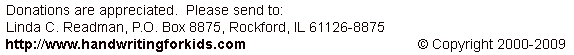Math: How to use the subtraction tableInstructions: 1. Pick a "pink" number on the left. 2. Pick a "blue" number on the top. 3. Move along the row from the "pink" number and down the column from the "blue" number to where they met for the answer. Example: 1. Pick a "pink" number on the left, example "6". 2. Pick a "blue" number on the top, example "3". 3. Move along the "6" row from the "pink" number and down the "3" column from the "blue" number to where they met at the "yellow" answer, example "= 3". (Follow the arrows to the "yellow" box.)